Nasty Polka Music

# npm

Learn about our RFC process, Open RFC meetings & more.Join in the discussion! »

## d3-geo

1.12.1 • Public • Published

# d3-geo

Map projections are sometimes implemented as point transformations. For instance, spherical Mercator:

This is a reasonable mathematical approach if your geometry consists of continuous, infinite point sets. Yet computers do not have infinite memory, so we must instead work with discrete geometry such as polygons and polylines!

Discrete geometry makes the challenge of projecting from the sphere to the plane much harder. The edges of a spherical polygon are geodesics (segments of great circles), not straight lines. Projected to the plane, geodesics are curves in all map projections except gnomonic, and thus accurate projection requires interpolation along each arc. D3 uses adaptive sampling inspired by a popular line simplification method to balance accuracy and performance.

The projection of polygons and polylines must also deal with the topological differences between the sphere and the plane. Some projections require cutting geometry that crosses the antimeridian, while others require clipping geometry to a great circle.

Spherical polygons also require a winding order convention to determine which side of the polygon is the inside: the exterior ring for polygons smaller than a hemisphere must be clockwise, while the exterior ring for polygons larger than a hemisphere must be anticlockwise. Interior rings representing holes must use the opposite winding order of their exterior ring. This winding order convention is also used by TopoJSON and ESRI shapefiles; however, it is the opposite convention of GeoJSON’s RFC 7946. (Also note that standard GeoJSON WGS84 uses planar equirectangular coordinates, not spherical coordinates, and thus may require stitching to remove antimeridian cuts.)

D3’s approach affords great expressiveness: you can choose the right projection, and the right aspect, for your data. D3 supports a wide variety of common and unusual map projections. For more, see Part 2 of The Toolmaker’s Guide.

D3 uses GeoJSON to represent geographic features in JavaScript. (See also TopoJSON, an extension of GeoJSON that is significantly more compact and encodes topology.) To convert shapefiles to GeoJSON, use shp2json, part of the shapefile package. See Command-Line Cartography for an introduction to d3-geo and related tools.

## Installing

If you use NPM, `npm install d3-geo`. Otherwise, download the latest release. You can also load directly from d3js.org, either as a standalone library or as part of D3. AMD, CommonJS, and vanilla environments are supported. In vanilla, a `d3` global is exported:

## API Reference

### Paths

The geographic path generator, d3.geoPath, is similar to the shape generators in d3-shape: given a GeoJSON geometry or feature object, it generates an SVG path data string or renders the path to a Canvas. Canvas is recommended for dynamic or interactive projections to improve performance. Paths can be used with projections or transforms, or they can be used to render planar geometry directly to Canvas or SVG.

# d3.geoPath([projection[, context]]) <>

Creates a new geographic path generator with the default settings. If projection is specified, sets the current projection. If context is specified, sets the current context.

# path(object[, arguments…]) <>

Renders the given object, which may be any GeoJSON feature or geometry object:

• Point - a single position.
• MultiPoint - an array of positions.
• LineString - an array of positions forming a continuous line.
• MultiLineString - an array of arrays of positions forming several lines.
• Polygon - an array of arrays of positions forming a polygon (possibly with holes).
• MultiPolygon - a multidimensional array of positions forming multiple polygons.
• GeometryCollection - an array of geometry objects.
• Feature - a feature containing one of the above geometry objects.
• FeatureCollection - an array of feature objects.

The type Sphere is also supported, which is useful for rendering the outline of the globe; a sphere has no coordinates. Any additional arguments are passed along to the pointRadius accessor.

To display multiple features, combine them into a feature collection:

Or use multiple path elements:

Separate path elements are typically slower than a single path element. However, distinct path elements are useful for styling and interaction (e.g., click or mouseover). Canvas rendering (see path.context) is typically faster than SVG, but requires more effort to implement styling and interaction.

# path.area(object) <>

Returns the projected planar area (typically in square pixels) for the specified GeoJSON object. Point, MultiPoint, LineString and MultiLineString geometries have zero area. For Polygon and MultiPolygon geometries, this method first computes the area of the exterior ring, and then subtracts the area of any interior holes. This method observes any clipping performed by the projection; see projection.clipAngle and projection.clipExtent. This is the planar equivalent of d3.geoArea.

# path.bounds(object) <>

Returns the projected planar bounding box (typically in pixels) for the specified GeoJSON object. The bounding box is represented by a two-dimensional array: [[x₀, y₀], [x₁, y₁]], where x₀ is the minimum x-coordinate, y₀ is the minimum y-coordinate, x₁ is maximum x-coordinate, and y₁ is the maximum y-coordinate. This is handy for, say, zooming in to a particular feature. (Note that in projected planar coordinates, the minimum latitude is typically the maximum y-value, and the maximum latitude is typically the minimum y-value.) This method observes any clipping performed by the projection; see projection.clipAngle and projection.clipExtent. This is the planar equivalent of d3.geoBounds.

# path.centroid(object) <>

Returns the projected planar centroid (typically in pixels) for the specified GeoJSON object. This is handy for, say, labeling state or county boundaries, or displaying a symbol map. For example, a noncontiguous cartogram might scale each state around its centroid. This method observes any clipping performed by the projection; see projection.clipAngle and projection.clipExtent. This is the planar equivalent of d3.geoCentroid.

# path.measure(object) <>

Returns the projected planar length (typically in pixels) for the specified GeoJSON object. Point and MultiPoint geometries have zero length. For Polygon and MultiPolygon geometries, this method computes the summed length of all rings. This method observes any clipping performed by the projection; see projection.clipAngle and projection.clipExtent. This is the planar equivalent of d3.geoLength.

# path.projection([projection]) <>

If a projection is specified, sets the current projection to the specified projection. If projection is not specified, returns the current projection, which defaults to null. The null projection represents the identity transformation: the input geometry is not projected and is instead rendered directly in raw coordinates. This can be useful for fast rendering of pre-projected geometry, or for fast rendering of the equirectangular projection.

The given projection is typically one of D3’s built-in geographic projections; however, any object that exposes a projection.stream function can be used, enabling the use of custom projections. See D3’s transforms for more examples of arbitrary geometric transformations.

# path.context([context]) <>

If context is specified, sets the current render context and returns the path generator. If the context is null, then the path generator will return an SVG path string; if the context is non-null, the path generator will instead call methods on the specified context to render geometry. The context must implement the following subset of the CanvasRenderingContext2D API:

• context.beginPath()
• context.moveTo(x, y)
• context.lineTo(x, y)
• context.arc(x, y, radius, startAngle, endAngle)
• context.closePath()

If a context is not specified, returns the current render context which defaults to null.

If radius is specified, sets the radius used to display Point and MultiPoint geometries to the specified number. If radius is not specified, returns the current radius accessor, which defaults to 4.5. While the radius is commonly specified as a number constant, it may also be specified as a function which is computed per feature, being passed the any arguments passed to the path generator. For example, if your GeoJSON data has additional properties, you might access those properties inside the radius function to vary the point size; alternatively, you could d3.symbol and a projection for greater flexibility.

### Projections

Projections transform spherical polygonal geometry to planar polygonal geometry. D3 provides implementations of several classes of standard projections:

For many more projections, see d3-geo-projection. You can implement custom projections using d3.geoProjection or d3.geoProjectionMutator.

# projection(point) <>

Returns a new array [x, y] (typically in pixels) representing the projected point of the given point. The point must be specified as a two-element array [longitude, latitude] in degrees. May return null if the specified point has no defined projected position, such as when the point is outside the clipping bounds of the projection.

# projection.invert(point) <>

Returns a new array [longitude, latitude] in degrees representing the unprojected point of the given projected point. The point must be specified as a two-element array [x, y] (typically in pixels). May return null if the specified point has no defined projected position, such as when the point is outside the clipping bounds of the projection.

This method is only defined on invertible projections.

# projection.stream(stream) <>

Returns a projection stream for the specified output stream. Any input geometry is projected before being streamed to the output stream. A typical projection involves several geometry transformations: the input geometry is first converted to radians, rotated on three axes, clipped to the small circle or cut along the antimeridian, and lastly projected to the plane with adaptive resampling, scale and translation.

# projection.preclip([preclip])

If preclip is specified, sets the projection’s spherical clipping to the specified function and returns the projection. If preclip is not specified, returns the current spherical clipping function (see preclip).

# projection.postclip([postclip])

If postclip is specified, sets the projection’s cartesian clipping to the specified function and returns the projection. If postclip is not specified, returns the current cartesian clipping function (see postclip).

# projection.clipAngle([angle]) <>

If angle is specified, sets the projection’s clipping circle radius to the specified angle in degrees and returns the projection. If angle is null, switches to antimeridian cutting rather than small-circle clipping. If angle is not specified, returns the current clip angle which defaults to null. Small-circle clipping is independent of viewport clipping via projection.clipExtent.

# projection.clipExtent([extent]) <>

If extent is specified, sets the projection’s viewport clip extent to the specified bounds in pixels and returns the projection. The extent bounds are specified as an array [[x₀, y₀], [x₁, y₁]], where x₀ is the left-side of the viewport, y₀ is the top, x₁ is the right and y₁ is the bottom. If extent is null, no viewport clipping is performed. If extent is not specified, returns the current viewport clip extent which defaults to null. Viewport clipping is independent of small-circle clipping via projection.clipAngle.

# projection.scale([scale]) <>

If scale is specified, sets the projection’s scale factor to the specified value and returns the projection. If scale is not specified, returns the current scale factor; the default scale is projection-specific. The scale factor corresponds linearly to the distance between projected points; however, absolute scale factors are not equivalent across projections.

# projection.translate([translate]) <>

If translate is specified, sets the projection’s translation offset to the specified two-element array [tx, ty] and returns the projection. If translate is not specified, returns the current translation offset which defaults to [480, 250]. The translation offset determines the pixel coordinates of the projection’s center. The default translation offset places ⟨0°,0°⟩ at the center of a 960×500 area.

# projection.center([center]) <>

If center is specified, sets the projection’s center to the specified center, a two-element array of [longitude, latitude] in degrees and returns the projection. If center is not specified, returns the current center, which defaults to ⟨0°,0°⟩.

# projection.angle([angle]) <>

If angle is specified, sets the projection’s post-projection planar rotation angle to the specified angle in degrees and returns the projection. If angle is not specified, returns the projection’s current angle, which defaults to 0°. Note that it may be faster to rotate during rendering (e.g., using context.rotate) rather than during projection.

# projection.reflectX([reflect])

If reflect is specified, sets whether or not the x-dimension is reflected (negated) in the output. If reflect is not specified, returns true if x-reflection is enabled, which defaults to false. This can be useful to display sky and astronomical data with the orb seen from below: right ascension (eastern direction) will point to the left when North is pointing up.

# projection.reflectY([reflect])

If reflect is specified, sets whether or not the y-dimension is reflected (negated) in the output. If reflect is not specified, returns true if y-reflection is enabled, which defaults to false. This is especially useful for transforming from standard spatial reference systems, which treat positive y as pointing up, to display coordinate systems such as Canvas and SVG, which treat positive y as pointing down.

# projection.rotate([angles]) <>

If rotation is specified, sets the projection’s three-axis spherical rotation to the specified angles, which must be a two- or three-element array of numbers [lambda, phi, gamma] specifying the rotation angles in degrees about each spherical axis. (These correspond to yaw, pitch and roll.) If the rotation angle gamma is omitted, it defaults to 0. See also d3.geoRotation. If rotation is not specified, returns the current rotation which defaults [0, 0, 0].

# projection.precision([precision]) <>

If precision is specified, sets the threshold for the projection’s adaptive resampling to the specified value in pixels and returns the projection. This value corresponds to the Douglas–Peucker distance. If precision is not specified, returns the projection’s current resampling precision which defaults to √0.5 ≅ 0.70710…

# projection.fitExtent(extent, object) <>

Sets the projection’s scale and translate to fit the specified GeoJSON object in the center of the given extent. The extent is specified as an array [[x₀, y₀], [x₁, y₁]], where x₀ is the left side of the bounding box, y₀ is the top, x₁ is the right and y₁ is the bottom. Returns the projection.

For example, to scale and translate the New Jersey State Plane projection to fit a GeoJSON object nj in the center of a 960×500 bounding box with 20 pixels of padding on each side:

Any clip extent is ignored when determining the new scale and translate. The precision used to compute the bounding box of the given object is computed at an effective scale of 150.

# projection.fitSize(size, object) <>

A convenience method for projection.fitExtent where the top-left corner of the extent is [0, 0]. The following two statements are equivalent:

# projection.fitWidth(width, object) <>

A convenience method for projection.fitSize where the height is automatically chosen from the aspect ratio of object and the given constraint on width.

# projection.fitHeight(height, object) <>

A convenience method for projection.fitSize where the width is automatically chosen from the aspect ratio of object and the given constraint on height.

#### Azimuthal Projections

Azimuthal projections project the sphere directly onto a plane.

# d3.geoAzimuthalEqualArea() <>
# d3.geoAzimuthalEqualAreaRaw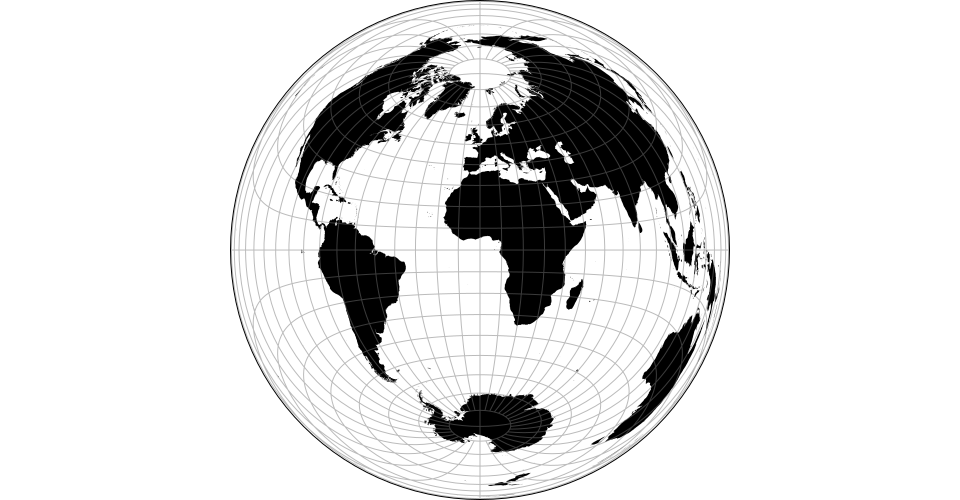The azimuthal equal-area projection.

# d3.geoAzimuthalEquidistant() <>
# d3.geoAzimuthalEquidistantRaw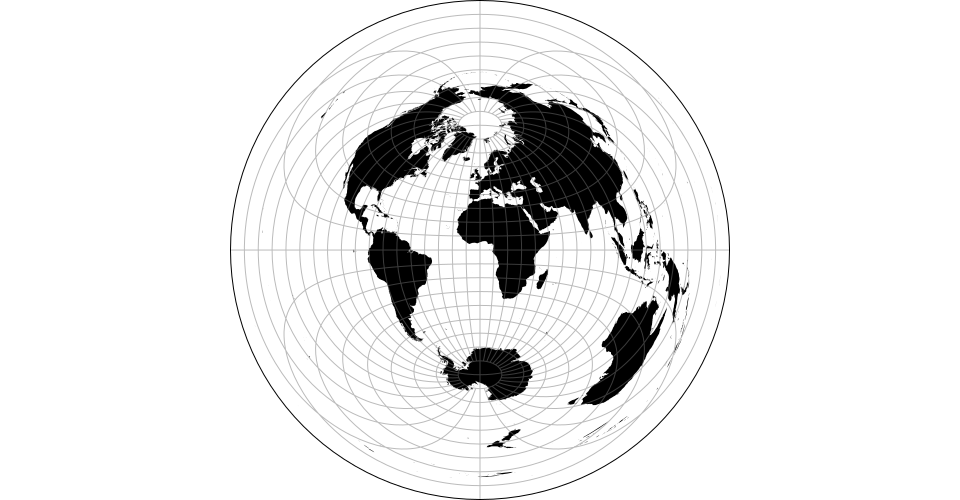The azimuthal equidistant projection.

# d3.geoGnomonic() <>
# d3.geoGnomonicRaw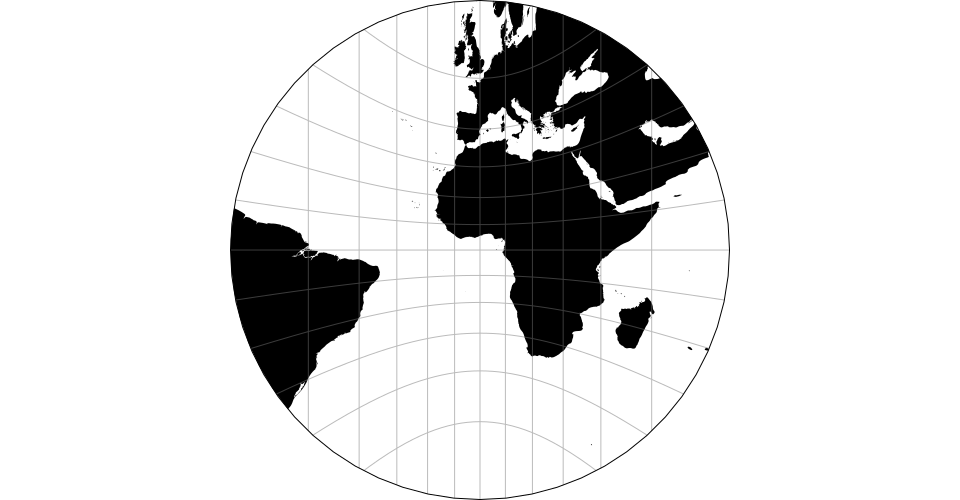The gnomonic projection.

# d3.geoOrthographic() <>
# d3.geoOrthographicRaw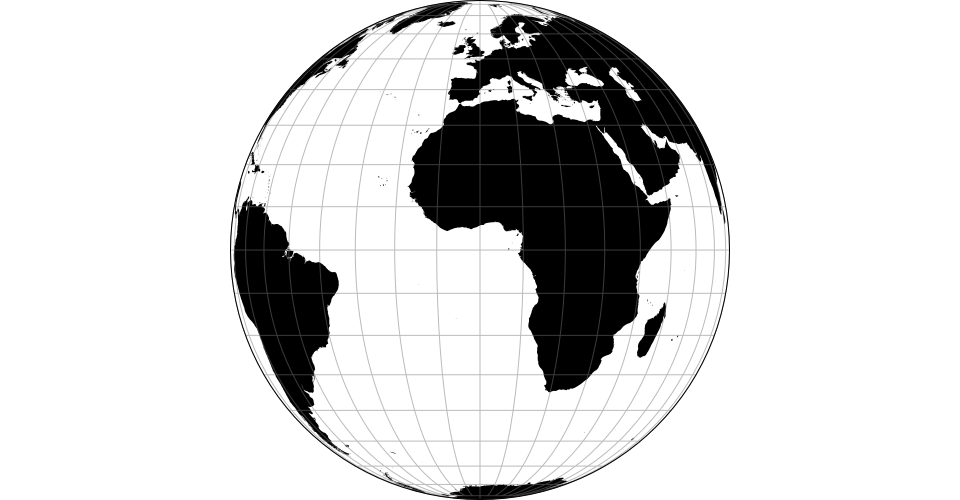The orthographic projection.

# d3.geoStereographic() <>
# d3.geoStereographicRaw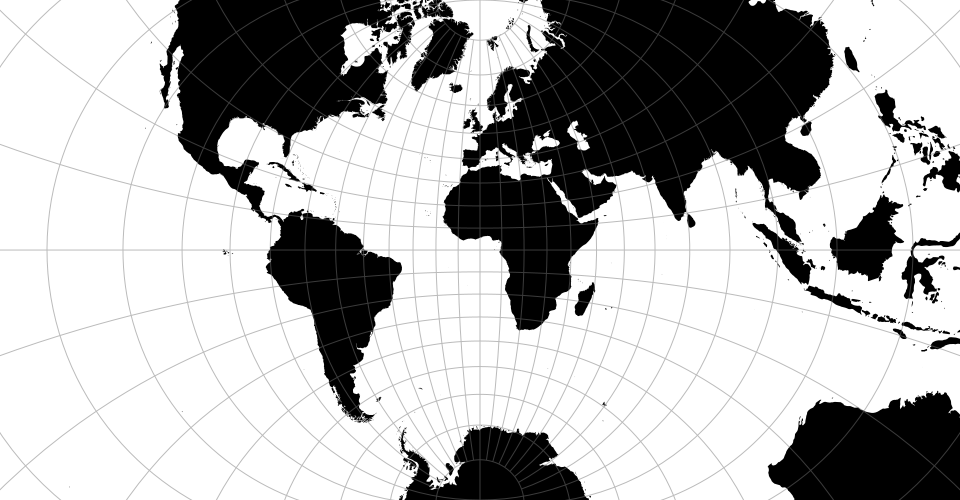The stereographic projection.

#### Equal-Earth

# d3.geoEqualEarth() <>
# d3.geoEqualEarthRaw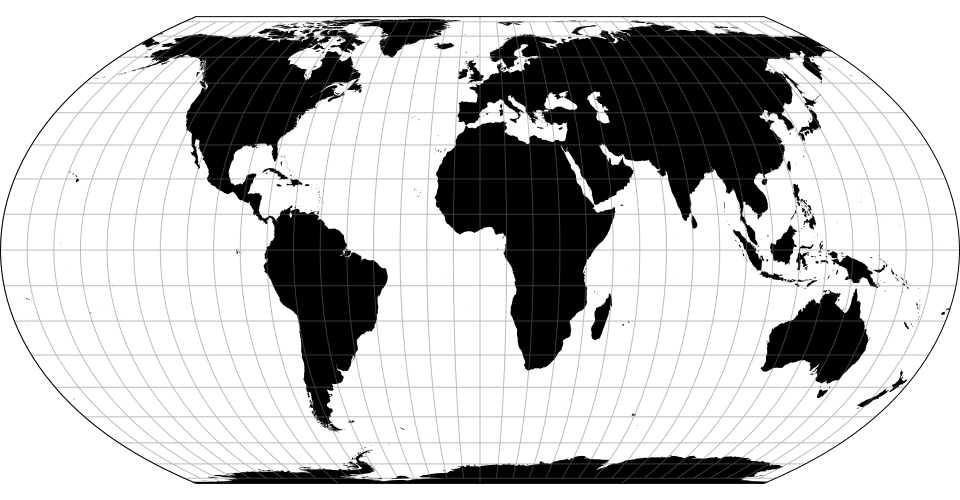The Equal Earth projection, by Bojan Šavrič et al., 2018.

#### Composite Projections

Composite consist of several projections that are composed into a single display. The constituent projections have fixed clip, center and rotation, and thus composite projections do not support projection.center, projection.rotate, projection.clipAngle, or projection.clipExtent.

# d3.geoAlbersUsa() <>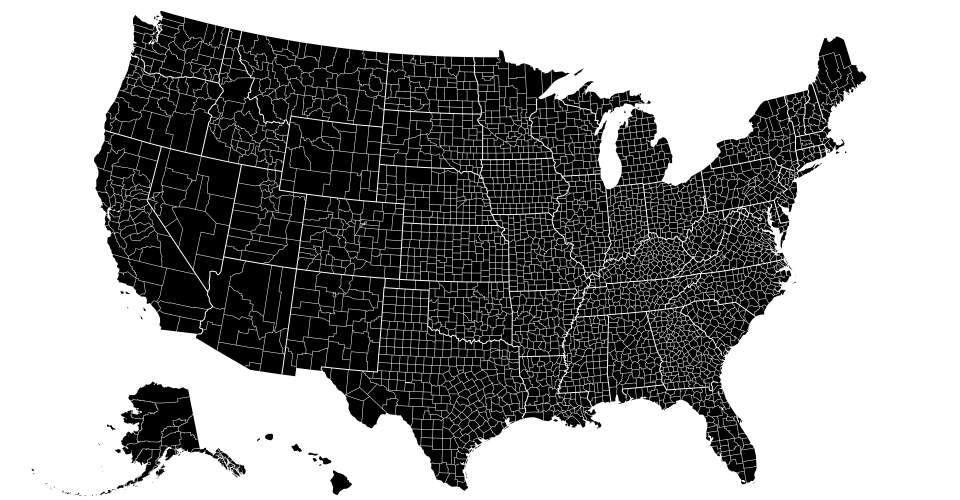This is a U.S.-centric composite projection of three d3.geoConicEqualArea projections: d3.geoAlbers is used for the lower forty-eight states, and separate conic equal-area projections are used for Alaska and Hawaii. Note that the scale for Alaska is diminished: it is projected at 0.35× its true relative area. This diagram by Philippe Rivière illustrates how this projection uses two rectangular insets for Alaska and Hawaii: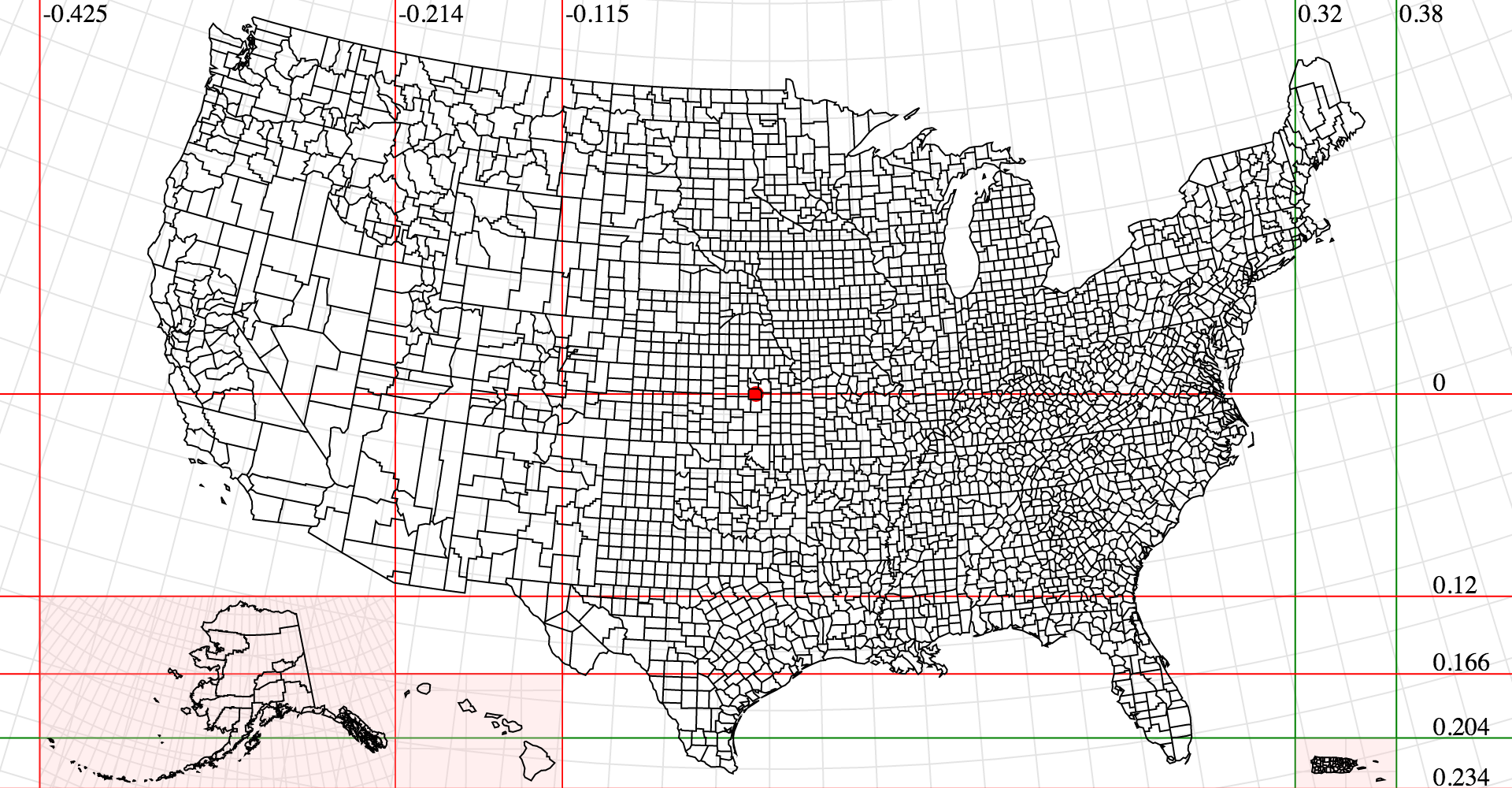See d3-composite-projections for more examples.

#### Conic Projections

Conic projections project the sphere onto a cone, and then unroll the cone onto the plane. Conic projections have two standard parallels.

# conic.parallels([parallels]) <>

The two standard parallels that define the map layout in conic projections.

# d3.geoAlbers() <>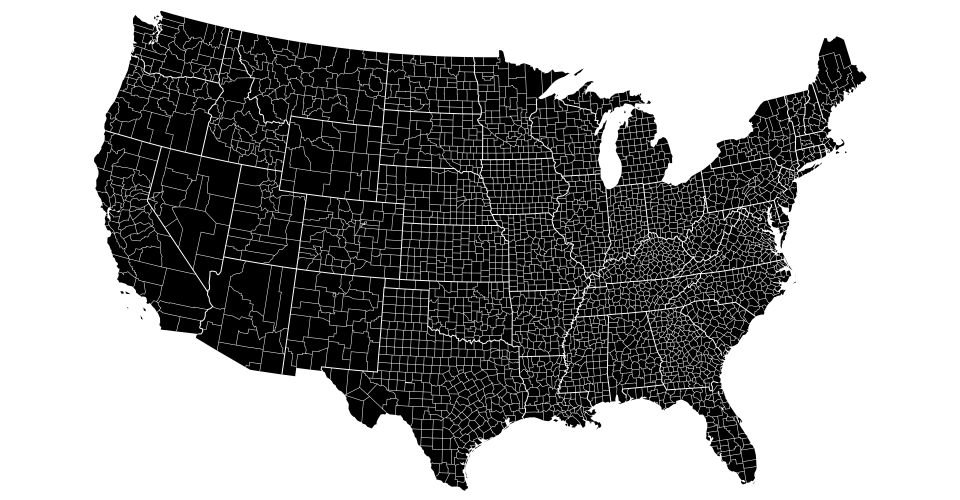The Albers’ equal area-conic projection. This is a U.S.-centric configuration of d3.geoConicEqualArea.

# d3.geoConicConformal() <>
# d3.geoConicConformalRaw(phi0, phi1) <>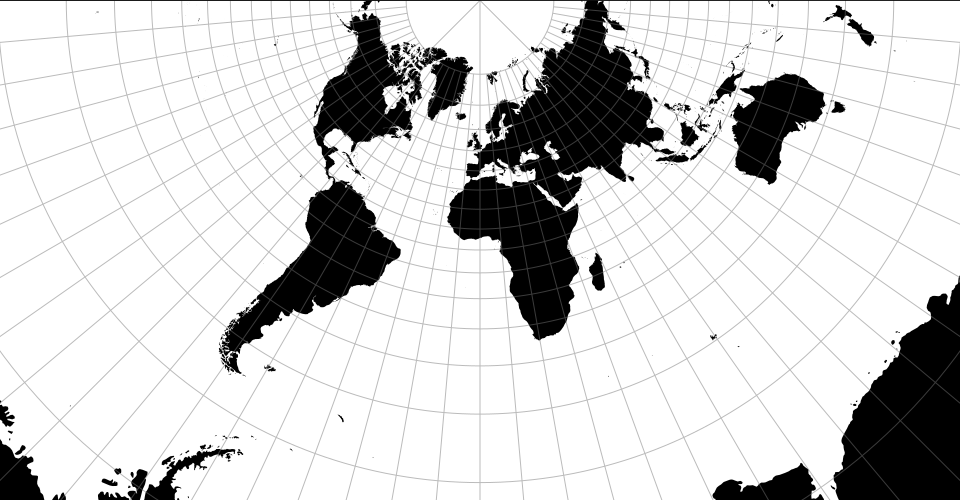The conic conformal projection. The parallels default to [30°, 30°] resulting in flat top. See also conic.parallels.

# d3.geoConicEqualArea() <>
# d3.geoConicEqualAreaRaw(phi0, phi1) <>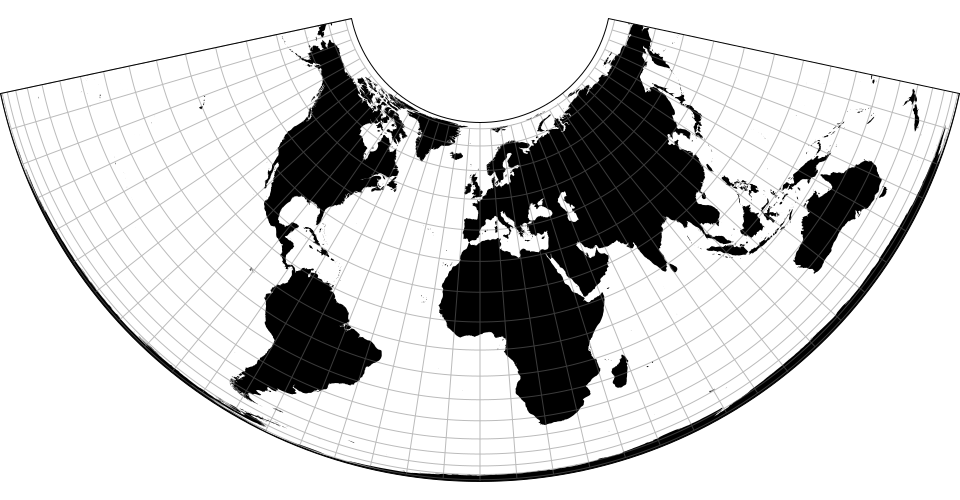# d3.geoConicEquidistant() <>
# d3.geoConicEquidistantRaw(phi0, phi1) <>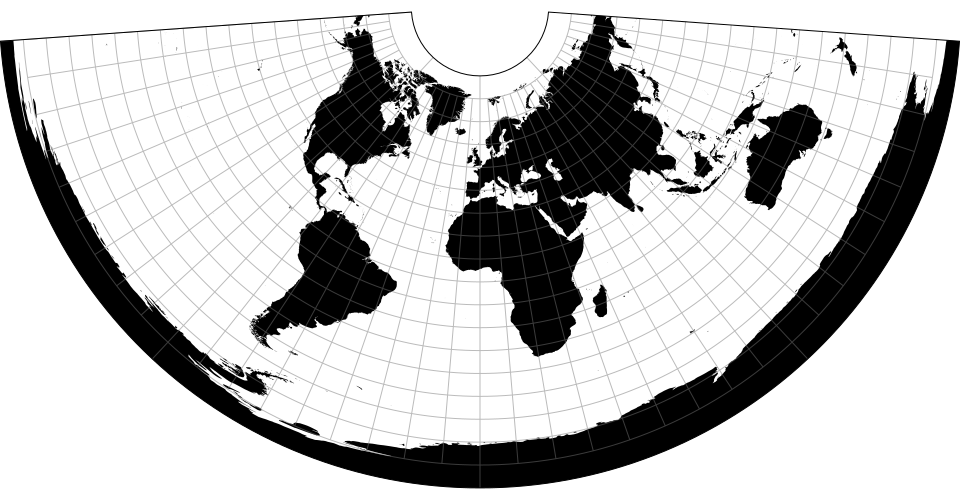#### Cylindrical Projections

Cylindrical projections project the sphere onto a containing cylinder, and then unroll the cylinder onto the plane. Pseudocylindrical projections are a generalization of cylindrical projections.

# d3.geoEquirectangular() <>
# d3.geoEquirectangularRaw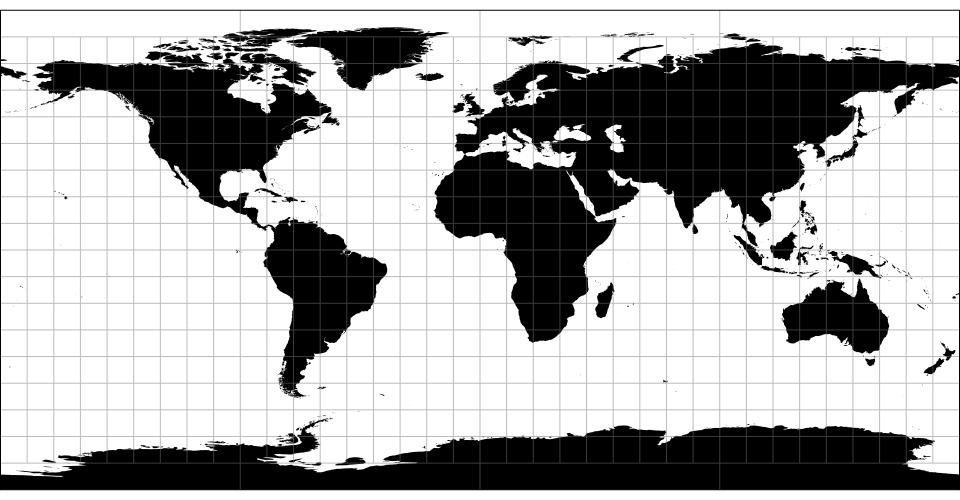The equirectangular (plate carrée) projection.

# d3.geoMercator() <>
# d3.geoMercatorRaw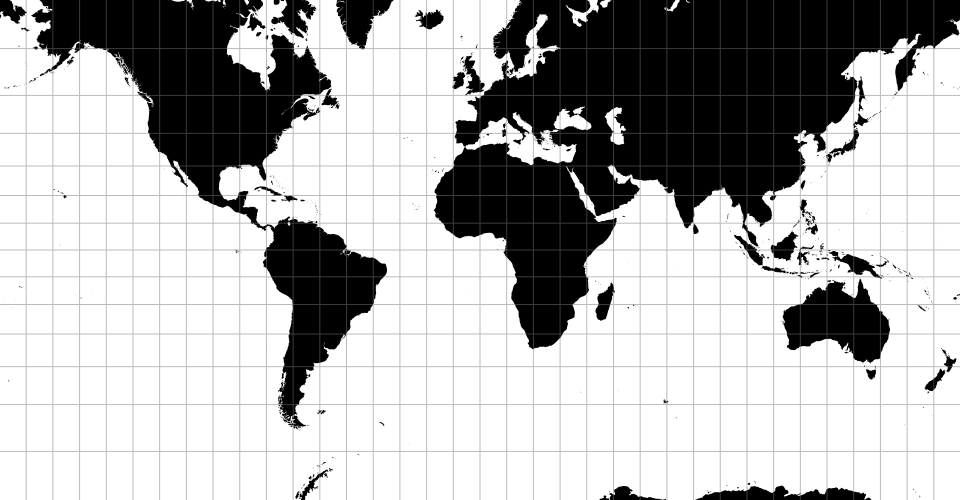The spherical Mercator projection. Defines a default projection.clipExtent such that the world is projected to a square, clipped to approximately ±85° latitude.

# d3.geoTransverseMercator() <>
# d3.geoTransverseMercatorRaw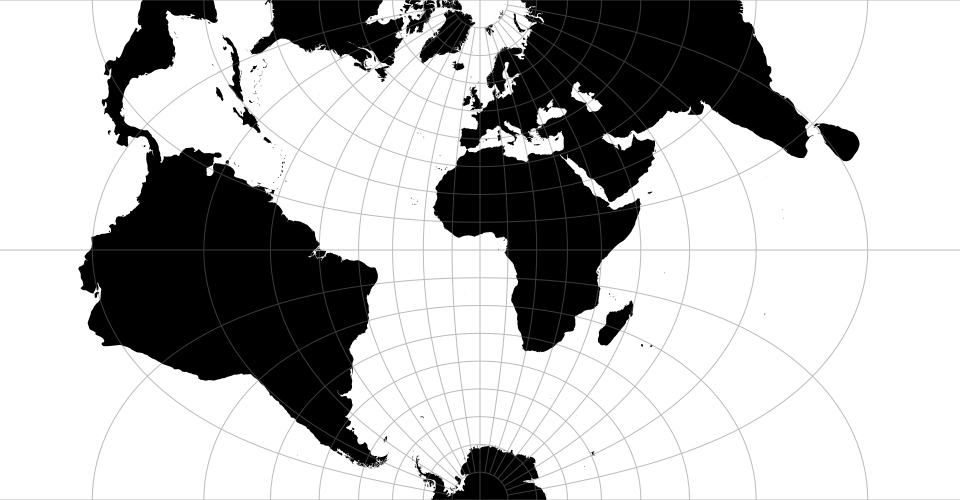The transverse spherical Mercator projection. Defines a default projection.clipExtent such that the world is projected to a square, clipped to approximately ±85° latitude.

# d3.geoNaturalEarth1() <>
# d3.geoNaturalEarth1Raw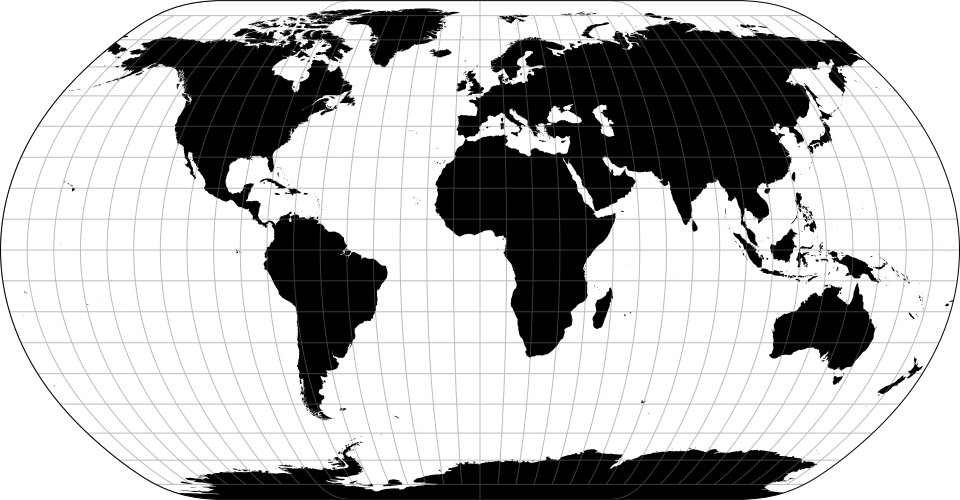The Natural Earth projection is a pseudocylindrical projection designed by Tom Patterson. It is neither conformal nor equal-area, but appealing to the eye for small-scale maps of the whole world.

### Raw Projections

Raw projections are point transformation functions that are used to implement custom projections; they typically passed to d3.geoProjection or d3.geoProjectionMutator. They are exposed here to facilitate the derivation of related projections. Raw projections take spherical coordinates [lambda, phi] in radians (not degrees!) and return a point [x, y], typically in the unit square centered around the origin.

# project(lambda, phi)

Projects the specified point [lambda, phi] in radians, returning a new point [x, y] in unitless coordinates.

# project.invert(x, y)

The inverse of project.

# d3.geoProjection(project) <>

Constructs a new projection from the specified raw projection, project. The project function takes the longitude and latitude of a given point in radians, often referred to as lambda (λ) and phi (φ), and returns a two-element array [x, y] representing its unit projection. The project function does not need to scale or translate the point, as these are applied automatically by projection.scale, projection.translate, and projection.center. Likewise, the project function does not need to perform any spherical rotation, as projection.rotate is applied prior to projection.

For example, a spherical Mercator projection can be implemented as:

If the project function exposes an invert method, the returned projection will also expose projection.invert.

# d3.geoProjectionMutator(factory) <>

Constructs a new projection from the specified raw projection factory and returns a mutate function to call whenever the raw projection changes. The factory must return a raw projection. The returned mutate function returns the wrapped projection. For example, a conic projection typically has two configurable parallels. A suitable factory function, such as d3.geoConicEqualAreaRaw, would have the form:

Using d3.geoProjectionMutator, you can implement a standard projection that allows the parallels to be changed, reassigning the raw projection used internally by d3.geoProjection:

When creating a mutable projection, the mutate function is typically not exposed.

### Spherical Math

# d3.geoArea(object) <>

Returns the spherical area of the specified GeoJSON object in steradians. This is the spherical equivalent of path.area.

# d3.geoBounds(object) <>

Returns the spherical bounding box for the specified GeoJSON object. The bounding box is represented by a two-dimensional array: [[left, bottom], [right, top]], where left is the minimum longitude, bottom is the minimum latitude, right is maximum longitude, and top is the maximum latitude. All coordinates are given in degrees. (Note that in projected planar coordinates, the minimum latitude is typically the maximum y-value, and the maximum latitude is typically the minimum y-value.) This is the spherical equivalent of path.bounds.

# d3.geoCentroid(object) <>

Returns the spherical centroid of the specified GeoJSON object. This is the spherical equivalent of path.centroid.

# d3.geoDistance(a, b) <>

Returns the great-arc distance in radians between the two points a and b. Each point must be specified as a two-element array [longitude, latitude] in degrees. This is the spherical equivalent of path.measure given a LineString of two points.

# d3.geoLength(object) <>

Returns the great-arc length of the specified GeoJSON object in radians. For polygons, returns the perimeter of the exterior ring plus that of any interior rings. This is the spherical equivalent of path.measure.

# d3.geoInterpolate(a, b) <>

Returns an interpolator function given two points a and b. Each point must be specified as a two-element array [longitude, latitude] in degrees. The returned interpolator function takes a single argument t, where t is a number ranging from 0 to 1; a value of 0 returns the point a, while a value of 1 returns the point b. Intermediate values interpolate from a to b along the great arc that passes through both a and b. If a and b are antipodes, an arbitrary great arc is chosen.

# d3.geoContains(object, point) <>

Returns true if and only if the specified GeoJSON object contains the specified point, or false if the object does not contain the point. The point must be specified as a two-element array [longitude, latitude] in degrees. For Point and MultiPoint geometries, an exact test is used; for a Sphere, true is always returned; for other geometries, an epsilon threshold is applied.

# d3.geoRotation(angles) <>

Returns a rotation function for the given angles, which must be a two- or three-element array of numbers [lambda, phi, gamma] specifying the rotation angles in degrees about each spherical axis. (These correspond to yaw, pitch and roll.) If the rotation angle gamma is omitted, it defaults to 0. See also projection.rotate.

# rotation(point) <>

Returns a new array [longitude, latitude] in degrees representing the rotated point of the given point. The point must be specified as a two-element array [longitude, latitude] in degrees.

# rotation.invert(point) <>

Returns a new array [longitude, latitude] in degrees representing the point of the given rotated point; the inverse of rotation. The point must be specified as a two-element array [longitude, latitude] in degrees.

### Spherical Shapes

To generate a great arc (a segment of a great circle), simply pass a GeoJSON LineString geometry object to a d3.geoPath. D3’s projections use great-arc interpolation for intermediate points, so there’s no need for a great arc shape generator.

# d3.geoCircle() <>

Returns a new circle generator.

# circle(arguments…) <>

Returns a new GeoJSON geometry object of type “Polygon” approximating a circle on the surface of a sphere, with the current center, radius and precision. Any arguments are passed to the accessors.

# circle.center([center]) <>

If center is specified, sets the circle center to the specified point [longitude, latitude] in degrees, and returns this circle generator. The center may also be specified as a function; this function will be invoked whenever a circle is generated, being passed any arguments passed to the circle generator. If center is not specified, returns the current center accessor, which defaults to:

If radius is specified, sets the circle radius to the specified angle in degrees, and returns this circle generator. The radius may also be specified as a function; this function will be invoked whenever a circle is generated, being passed any arguments passed to the circle generator. If radius is not specified, returns the current radius accessor, which defaults to:

# circle.precision([angle]) <>

If precision is specified, sets the circle precision to the specified angle in degrees, and returns this circle generator. The precision may also be specified as a function; this function will be invoked whenever a circle is generated, being passed any arguments passed to the circle generator. If precision is not specified, returns the current precision accessor, which defaults to:

Small circles do not follow great arcs and thus the generated polygon is only an approximation. Specifying a smaller precision angle improves the accuracy of the approximate polygon, but also increase the cost to generate and render it.

# d3.geoGraticule() <>

Constructs a geometry generator for creating graticules: a uniform grid of meridians and parallels for showing projection distortion. The default graticule has meridians and parallels every 10° between ±80° latitude; for the polar regions, there are meridians every 90°.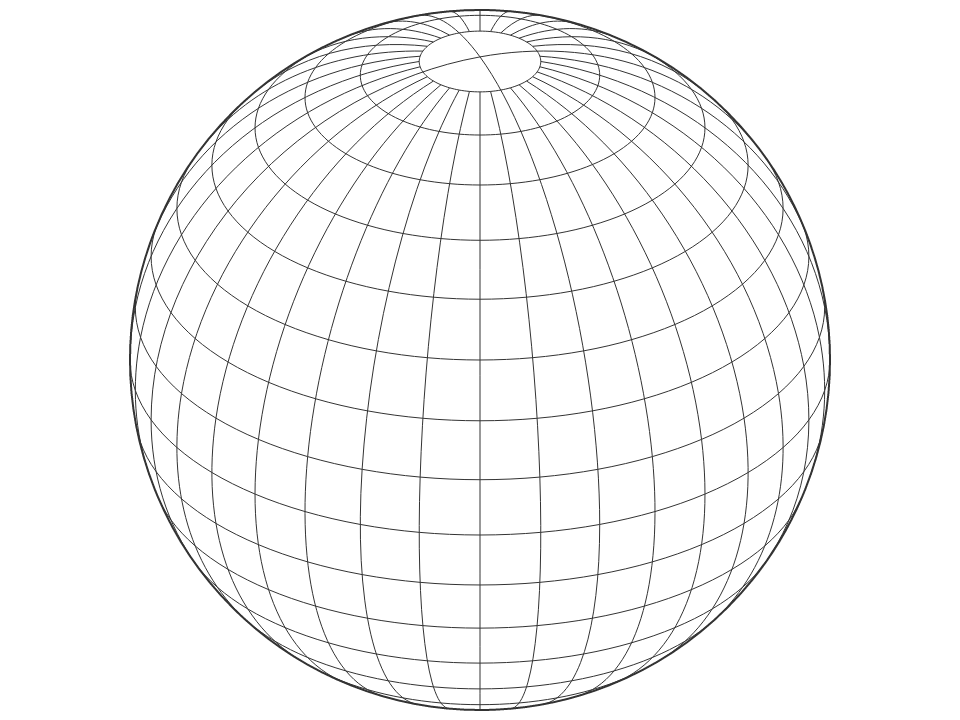# graticule() <>

Returns a GeoJSON MultiLineString geometry object representing all meridians and parallels for this graticule.

# graticule.lines() <>

Returns an array of GeoJSON LineString geometry objects, one for each meridian or parallel for this graticule.

# graticule.outline() <>

Returns a GeoJSON Polygon geometry object representing the outline of this graticule, i.e. along the meridians and parallels defining its extent.

# graticule.extent([extent]) <>

If extent is specified, sets the major and minor extents of this graticule. If extent is not specified, returns the current minor extent, which defaults to ⟨⟨-180°, -80° - ε⟩, ⟨180°, 80° + ε⟩⟩.

# graticule.extentMajor([extent]) <>

If extent is specified, sets the major extent of this graticule. If extent is not specified, returns the current major extent, which defaults to ⟨⟨-180°, -90° + ε⟩, ⟨180°, 90° - ε⟩⟩.

# graticule.extentMinor([extent]) <>

If extent is specified, sets the minor extent of this graticule. If extent is not specified, returns the current minor extent, which defaults to ⟨⟨-180°, -80° - ε⟩, ⟨180°, 80° + ε⟩⟩.

# graticule.step([step]) <>

If step is specified, sets the major and minor step for this graticule. If step is not specified, returns the current minor step, which defaults to ⟨10°, 10°⟩.

# graticule.stepMajor([step]) <>

If step is specified, sets the major step for this graticule. If step is not specified, returns the current major step, which defaults to ⟨90°, 360°⟩.

# graticule.stepMinor([step]) <>

If step is specified, sets the minor step for this graticule. If step is not specified, returns the current minor step, which defaults to ⟨10°, 10°⟩.

# graticule.precision([angle]) <>

If precision is specified, sets the precision for this graticule, in degrees. If precision is not specified, returns the current precision, which defaults to 2.5°.

# d3.geoGraticule10() <>

A convenience method for directly generating the default 10° global graticule as a GeoJSON MultiLineString geometry object. Equivalent to:

### Streams

D3 transforms geometry using a sequence of function calls, rather than materializing intermediate representations, to minimize overhead. Streams must implement several methods to receive input geometry. Streams are inherently stateful; the meaning of a point depends on whether the point is inside of a line, and likewise a line is distinguished from a ring by a polygon. Despite the name “stream”, these method calls are currently synchronous.

# d3.geoStream(object, stream) <>

Streams the specified GeoJSON object to the specified projection stream. While both features and geometry objects are supported as input, the stream interface only describes the geometry, and thus additional feature properties are not visible to streams.

# stream.point(x, y[, z])

Indicates a point with the specified coordinates x and y (and optionally z). The coordinate system is unspecified and implementation-dependent; for example, projection streams require spherical coordinates in degrees as input. Outside the context of a polygon or line, a point indicates a point geometry object (Point or MultiPoint). Within a line or polygon ring, the point indicates a control point.

# stream.lineStart()

Indicates the start of a line or ring. Within a polygon, indicates the start of a ring. The first ring of a polygon is the exterior ring, and is typically clockwise. Any subsequent rings indicate holes in the polygon, and are typically counterclockwise.

# stream.lineEnd()

Indicates the end of a line or ring. Within a polygon, indicates the end of a ring. Unlike GeoJSON, the redundant closing coordinate of a ring is not indicated via point, and instead is implied via lineEnd within a polygon. Thus, the given polygon input:

Will produce the following series of method calls on the stream:

# stream.polygonStart()

Indicates the start of a polygon. The first line of a polygon indicates the exterior ring, and any subsequent lines indicate interior holes.

# stream.polygonEnd()

Indicates the end of a polygon.

# stream.sphere()

Indicates the sphere (the globe; the unit sphere centered at ⟨0,0,0⟩).

### Transforms

Transforms are a generalization of projections. Transform implement projection.stream and can be passed to path.projection. However, they only implement a subset of the other projection methods, and represent arbitrary geometric transformations rather than projections from spherical to planar coordinates.

# d3.geoTransform(methods) <>

Defines an arbitrary transform using the methods defined on the specified methods object. Any undefined methods will use pass-through methods that propagate inputs to the output stream. For example, to reflect the y-dimension (see also identity.reflectY):

Or to define an affine matrix transformation:

# d3.geoIdentity() <>

The identity transform can be used to scale, translate and clip planar geometry. It implements projection.scale, projection.translate, projection.fitExtent, projection.fitSize, projection.fitWidth, projection.fitHeight, projection.clipExtent, projection.angle, projection.reflectX and projection.reflectY.

### Clipping

Projections perform cutting or clipping of geometries in two stages.

# preclip(stream)

Pre-clipping occurs in geographic coordinates. Cutting along the antimeridian line, or clipping along a small circle are the most common strategies.

# postclip(stream)

Post-clipping occurs on the plane, when a projection is bounded to a certain extent such as a rectangle.

Clipping functions are implemented as transformations of a projection stream. Pre-clipping operates on spherical coordinates, in radians. Post-clipping operates on planar coordinates, in pixels.

# d3.geoClipAntimeridian

A clipping function which transforms a stream such that geometries (lines or polygons) that cross the antimeridian line are cut in two, one on each side. Typically used for pre-clipping.

# d3.geoClipCircle(angle)

Generates a clipping function which transforms a stream such that geometries are bounded by a small circle of radius angle around the projection’s center. Typically used for pre-clipping.

# d3.geoClipRectangle(x0, y0, x1, y1)

Generates a clipping function which transforms a stream such that geometries are bounded by a rectangle of coordinates [[x0, y0], [x1, y1]]. Typically used for post-clipping.

## Keywords

### Install

`npm i d3-geo`

999,012

1.12.1

BSD-3-Clause

284 kB

70

### Homepage

d3js.org/d3-geo/

### Repository

github.com/d3/d3-geo